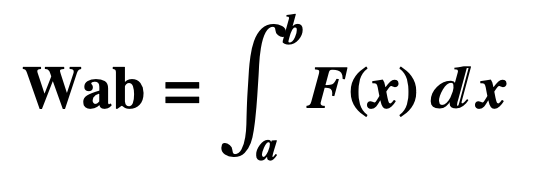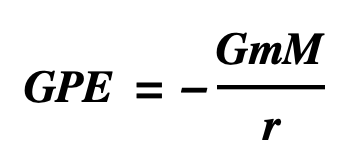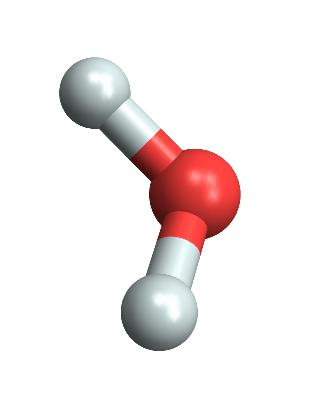# Theory of Energy

Back to Energy

### Work

• Energy is the capacity for doing work.
• Work is the energy transferred as a force moves an object
• britannica.com/science/work-physics
• “Work is the measure of energy transfer that occurs when an object is moved over a distance by an external force. If the force is constant, work may be computed by multiplying the length of the path by the magnitude of the force.”
• “Work done on a body is equal to the increase in the energy of the body, for work transfers energy to the body.}
• “Work and energy are expressed in the same units, for example, joules and foot-pounds.”
• Formal Definition
• Work by a force on an object over a distance is the force exerted times the distance covered.
• For a constant force in a straight line
• W = F x D
• For a variable force in a straight line from a to b
•• Dropping a bowling ball
• If a sixteen pound bowling ball is dropped five feet, Earth’s gravity does work on the ball equalling:
• 16 pounds of force x 5 feet travelled = 80 foot-pounds of work.
• The cue ball, with 10.6 joules of kinetic energy, hits a stationary eight ball:
• Before impact:
• Cue ball has kinetic energy of 10.6 joules
• Eight ball has zero.
• At Impact:
• 10.6 joules of work is performed, transferring 10.6 joules of energy from the cue ball to the eight ball
• After impact:
• The cue ball has zero kinetic energy
• Eight ball has kinetic energy of 10.6 joules
• The formula W = F x D is used to derive formulas for different forms of energy
• To derive the formula for kinetic energy, for example, you calculate the amount of work required to move a mass m from rest to v meters per second.
• That amount of work is ½ mv2 joules.

### Forms of Energy

###### The Forms
• Total energy
• The total energy of a system is the sum of the forms of energy in the system, each calculated by its formula.
• Forms of energy include
• Chemical energy
• Elastic potential energy
• Electrical energy
• Gravitational potential energy
• Kinetic energy
• Mass energy
• Nuclear energy
• Thermal energy

### The Two Basic Forms of Energy

• Kinetic Energy, the energy of motion
• The kinetic energy of an object moving at a given speed is the work required to accelerate the object from rest to that speed.
• KE = ½ mv2 is derived from W = FD and F = MA
• Potential Energy, the energy of location (in a force field)
• The potential energy of an object at a given location is the work required to move the object from a reference point to that location.
• For example, the formula for gravitational potential energy,
•• is derived from W = FD and Newton’s Law of Universal Gravitation.
• Force and potential energy are intimately connected:
• Force = change of potential energy divided by distance
• For example, electric forces among atoms bend water molecules
•• In the diagram the forces push downhill, from higher potential energy to lower.

### Potential and Kinetic Energy of the Pendulum

• The total energy of a pendulum system at a time = the kinetic energy of the bob at the time + the gravitational potential energy of the system at the time.
• The kinetic energy of the bob, calculated by ½ mv2, changes from zero to the total energy as the bob goes from its high point to its low point
• m = mass of bob
• v = velocity of bob
• The gravitational potential energy, calculated by gmh, changes from the total energy to zero as the bob goes from its high point to its low point
• g = acceleration due to gravity
• m = mass of bob
• h = height of bob

### Work and Heat:Forms of Energy Transfer,Not Forms of Energy

• Work is a form of energy transfer, not a form of energy
• Work is the energy transferred resulting from a force moving an object
• Heat is a form of energy transfer, not a form of energy
• Heat is the energy transferred resulting from a difference in temperature
• The total energy of a system does not include work and heat.
• Water Bucket Analogy
• A first bucket has five quarts of water and a second two.  You pour one quart from the first bucket into the second. The total amount of water, seven quarts, remains the same but it’s now divided differently: 4 + 3 vs 5 + 2.
• The seven quarts does not include the one quart transferred.

### Law of Conservation of Energy,Emmy Noether’s Proof

• In 1915 Emmy Noether (EM-mee ΝEW-tar) proved Noether’s Theorem, which says:
• If the laws of physics remain valid through transformation X, then quantity Y is conserved, where
• X = translations in time and Y = energy
• X = translations in space and Y = linear momentum
• X = rotations in space and Y = angular momentum
• X = gauge transformations and Y = electric charge
• Thus, Noether proved that the Law of the Conservation of Energy follows from the fact that the laws of physics remain valid through time.

### Conservation of Energy in Classical and Quantum Mechanics

• Proof of Conservation of Energy in Classical Mechanics
• E = ½ mv2  + V(x)
• The total energy of a system = kinetic energy + potential energy
• [several steps of reasoning]
• Therefore, dE/dt = 0
• The rate of change of the total energy of the system = 0
• Proof of Conservation of Energy in Quantum Mechanics
• For any operator Q, d/dt ⟨Q⟩ = -i/ℏ ⟨[Q,H]⟩, where H represents the total energy of a system
• The time rate of change of the expectation of an operator Q equals -i/ℏ times the expectation of the commutator of Q with H
• [H,H] = 0
• Every operator commutes with itself
• Therefore,  d/dt ⟨H⟩ = -i/ℏ ⟨[H,H]⟩ = 0
• The rate of change of the expectation of the Hamiltonian = 0